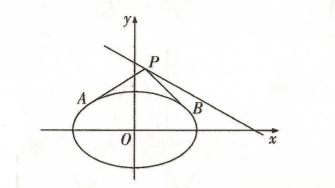(1) 若点 $P$ 为直线 $l$ 与 $y$ 轴的交点,求 $\triangle P A B$ 的面积 $S$;
(2)若 $O D \perp A B, D$ 为垂足, 求证: 存在定点 $Q$, 使得 $|D Q|$ 为定值.
【答案】 (1) 由题意知 $P(0,3)$, 过点 $P$ 与椭圆相切的直线料率存在, 设切线方程为 $y=k x+3$, 联立 $\left\{\begin{array}{l}y=k x+3, \\ x^2+2 y^2=6,\end{array}\right.$ 可得 $\left(2 k^2+1\right) x^2+12 k x+12=0,(*)$ 由 $\Delta=144 k^2-48\left(2 k^2+1\right)=48\left(k^2-1\right)=0$,

(2) 证明: 先证明出椭圆 $\frac{x^2}{6}+\frac{y^2}{3}=1$ 在其上一点 $M\left(x_0, y_0\right)$ 处的切线方程为 $\frac{x_0 x}{6}+\frac{y_0 y}{3}=1$,